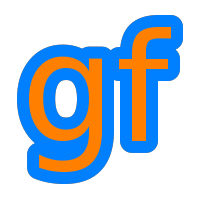Gamedev Framework (gf)  0.1.0 A C++11 framework for 2D games
Class Index
A | B | C | D | E | F | G | I | K | L | M | N | O | P | Q | R | S | T | V | W
 A
Direction (gf)
 K
 P
StarShape (gf)
Drawable (gf)   StretchView (gf)
Action (gf)
 E
Keyboard (gf)   PerlinNoise (gf)   SystemInfo (gf)
ActionContainer (gf)   KeycodeKeyControl (gf)   PostProcessing (gf)
 T
AdaptativeView (gf)   EdgeEffect (gf)   Event::KeyEvent (gf)
 Q
AlphaTexture (gf)   Effect (gf)
 L
Text (gf)
AnimatedSprite (gf)   Entity (gf)   QuadraticBezierCurve (gf)   Event::TextEvent (gf)
Animation (gf)   EntityContainer (gf)   Library (gf)   Queue (gf)   Texture (gf)
AntiAliasingEffect (gf)   Event (gf)   Line (gf)
 R
TextureAtlas (gf)
Array2D (gf)   ExtendView (gf)   Log (gf)   TileLayer (gf)
AssetManager (gf)
 F
 M
Random (gf)   Time (gf)
 B
Range (gf)   Transformable (gf)
FileInputStream (gf)   Matrix (gf)   Rect (gf)
 V
BareTexture (gf)   FillView (gf)   Matrix< T, 2, 2 > (gf)   RectangleShape (gf)
BlendMode (gf)   FitView (gf)   Matrix< T, 3, 3 > (gf)   RenderPipeline (gf)   Vector (gf)
BufferedGeometry (gf)   Font (gf)   Matrix< T, 4, 4 > (gf)   RenderStates (gf)   Vector< T, 2 > (gf)
 C
FractalNoise (gf)   MemoryInputStream (gf)   RenderTarget (gf)   Vector< T, 3 > (gf)
 G
Message (gf)   RenderTexture (gf)   Vector< T, 4 > (gf)
CircleShape (gf)   MessageManager (gf)   RenderWindow (gf)   Library::Version (gf)
Clock (gf)   Gamepad (gf)   Model (gf)   ResourceCache (gf)   Vertex (gf)
CloseControl (gf)   GamepadAxisControl (gf)   ModelContainer (gf)   ResourceManager (gf)   VertexArray (gf)
Color (gf)   Event::GamepadAxisEvent (gf)   Modifiers (gf)   RoundedRectangleShape (gf)   VertexBuffer (gf)
ColorBlindEffect (gf)   GamepadButtonControl (gf)   Monitor (gf)
 S
VideoMode (gf)
ColorEffect (gf)   Event::GamepadButtonEvent (gf)   MouseButtonControl (gf)   View (gf)
ColorMatrixEffect (gf)   Event::GamepadConnection (gf)   Event::MouseButtonEvent (gf)   ScancodeKeyControl (gf)   ViewContainer (gf)
ColorRamp (gf)   Event::GamepadDisconnection (gf)   Event::MouseCursorEvent (gf)   ScreenView (gf)
 W
CompoundCurve (gf)   Glyph (gf)   Event::MouseWheelEvent (gf)   Shader (gf)
 N
Shape (gf)   Window (gf)
ConvexShape (gf)
 I
SimplexNoise (gf)   WindowHints (gf)
CubicBezierCurve (gf)   NinePatch (gf)   Singleton (gf)
Curve (gf)   Image (gf)
 O
SingletonStorage (gf)
 D
InputStream (gf)   Sprite (gf)
Range::Iterator (gf)   OpenSimplexNoise (gf)   SpriteBatch (gf)
DefaultEffect (gf)
A | B | C | D | E | F | G | I | K | L | M | N | O | P | Q | R | S | T | V | W